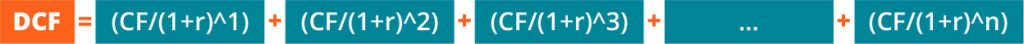The Projected DCF uses the cash flows from your projection scenarios to build a Discounted Cash Flow (DCF) valuation model.

The discounted cash flow (DCF) formula is equal to the sum of the cash flow in each period divided by one plus the discount rate (WACC) raised to the power of the period number.

Here is the DCF formula:Where:

CF = Cash Flow in the Period

r = the interest rate or discount rate

n = the period number

### Analyzing the components of the formula

#### Cash Flow (CF)

Cash Flow (CF) represents the free cash payments an investor receives in a given period for owning a given security (bonds, shares, etc.)

When building a financial model of a company, the CF is typically what’s known as unlevered free cash flow.  When valuing a bond, the CF would be interest and or principal payments.

#### Discount Rate (r)

For business valuation purposes, the discount rate is typically a firm’s Weighted Average Cost of Capital(WACC).  Investors use WACC because it represents the required rate of return that investors expect from investing in the company.

For a bond, the discount rate would be equal to the interest rate on the security.

#### Period Number (n)

Each cash flow is associated with a time period. Common time periods are years, quarters or months.  The time periods may be equal, or they may be different.  If they’re different, they’re expressed as a decimal.

### Terminal value

When valuing a business, the forecasted cash flow typically extends about 5 - 10 years into the future, at which point a terminal value is used.  The reason is that it becomes hard to make a reliable estimate of how a business will perform that far in the future.

There are two common methods of calculating the terminal value:

• Exit multiple (where the business is assumed to be sold)
• Perpetual growth (where the business is assumed to grow at a reasonable, fixed growth rate forever)

The Provamark model uses the Exit multiple approach.